The binomial distribution is the probability distribution for the number of successes in a sequence of Bernoulli trials. In $$n$$ Bernoulli trials the number of outcomes that contain exactly $$x$$ successes equals the binomial coefficient $${n \choose x}$$ (Weiss 2010).

Let $$X$$ denote the total number of successes in $$n$$ Bernoulli trials with the success probability $$p$$. Then the probability distribution of the random variable $$X$$ is given by:

$P(X = x) = {n \choose x}p^x(1 - p)^{n-x}, \qquad x = 0, 1, 2, . . . , n$

The random variable $$X$$ is called a binomial random variable and is said to have the binomial distribution with parameters $$n$$ and $$p$$ (Weiss 2010).

Let us consider an example taken form the real world.

Long term statistics tell us, that the chance for passing the final statistics exam is 0.3. Yes, approximately 70 % of students fail in their statistics exam! By the way, if you complete this e-learning module, your chances of passing the final exam will definitely increase :-)

Let us consider a class of 25 students. What is the chance that exactly 3 students of that particular class will pass the final statistics exam? Or written more formally $$P(X = 3)$$. Once again, we start by implementing a naive approach in R.

n <- 25 # number of students
p <- 0.3 # probability of success
k <- 3 # exactly 3 students will pass the exam

choose(n, k) * p^k * (1 - p)^(n - k)
##  0.02427999

Wow, the probability that exactly 3 out of 25 students $$(P(X = 3))$$ will pass the final statistics exam is quite low. What about the probability that exactly 15 out of 25 students $$(P(X = 15))$$ will pass the final statistics exam? We turn to R.

n <- 25 # number of students
p <- 0.3 # probability of success
k <- 15 # exactly 15 students will pass the exam

choose(n, k) * p^k * (1 - p)^(n - k)
##  0.001324897

The probability of $$P(X = 15)$$ is approximately 0.1 %. We can continue with our experiments to find out all the probabilities of exactly one outcome for $$k = 0,1,2,...,n$$. Please note, that for our particular example it is not very informative to know the probability of exactly one particular number ($$k$$) of students passing the exam. For us it is of higher informational interest if we can answer the question, what the probability that $$k$$ or less students $$(P(X \le k))$$ will pass the exam is or, equally interesting, that $$k$$ or more students $$(P(X \ge k))$$ will pass the exam.

As an exercise we turn to R and determine the probability that 9 or less students will pass the final statistics exam $$(P(X \le 9))$$. So, we are interested in the probability that 0 out of 25, or 1 out of 25, or 2 out of 25, …, or 9 out of 25 students will pass the exam. To calculate this probability we can extend our naive approach and calculate $$P(X = 0)+P(X = 1)+P(X = 2) + ... + P(X = 9)$$.

n <- 25 # number of students
p <- 0.3 # probability of success

choose(n, 0) * p^0 * (1 - p)^(n - 0) +
choose(n, 1) * p^1 * (1 - p)^(n - 1) +
choose(n, 2) * p^2 * (1 - p)^(n - 2) +
choose(n, 3) * p^3 * (1 - p)^(n - 3) +
choose(n, 4) * p^4 * (1 - p)^(n - 4) +
choose(n, 5) * p^5 * (1 - p)^(n - 5) +
choose(n, 6) * p^6 * (1 - p)^(n - 6) +
choose(n, 7) * p^7 * (1 - p)^(n - 7) +
choose(n, 8) * p^8 * (1 - p)^(n - 8) +
choose(n, 9) * p^9 * (1 - p)^(n - 9)
##  0.810564

The probability that 9 or less students will pass the statistics exam $$(P(X\le 9))$$ is 81.1 %. In turn, that means the probability of 10 or more students passing the exam is $$P(X > 9)= 1-P(X \le 9)$$ and only 18.9 %.

Exercise: Since it is quite a lot of typing, try to do the previous calculation based on a loop! (There are several ways to solve this exercise)

### your code here
Show code
n <- 25 # number of students
p <- 0.3 # probability of success

result <- 0
for (k in 0:9) {
result <- result + choose(n, k) * p^k * (1 - p)^(n - k)
}
result
##  0.810564

Beside the honestly quite unpleasant insight into the statistics of passing the final exam, it is clear that the naive implementation from above is quite tedious. Therefore, we introduce the in-built functions dbinom() and pbinom(). Call the help() on those functions for further information.

According to the help() function the usage of dbinom() is as follows: dbinom(x, size, prob, log = FALSE). We disregard the log argument and stay with its default log = FALSE. Thus, the dbinom() function simplifies to dbinom(x, size, prob), where x corresponds to our $$k$$, size to our $$n$$ and prob to our $$p$$. In order to check if the dbinom() function works as expected, we recall our test examples from above, which were $$P(X = 3)$$ and $$P(X = 15)$$.

n <- 25 # number of students
p <- 0.3 # probability of success
k <- c(3, 15)

dbinom(k, n, p)
##  0.02427999
dbinom(k, n, p)
##  0.001324897

Compare these results with the results of our naive implementation (scroll up). The numbers should match.

The dbinom() function becomes very handy, if we consider accumulated probabilities, such as $$P(X \le x)$$ or $$P(X > x)$$. We can specify a vector for the argument x and sum it up afterwards. Let us consider the example from above $$(P(X \le 9))$$.

n <- 25 # number of students
p <- 0.3 # probability of success
k <- 0:9

dbinom(k, n, p)
##   0.0001341069 0.0014368592 0.0073895618 0.0242799887 0.0572314020
##   0.1030165235 0.1471664622 0.1711936397 0.1650795811 0.1336358514
sum(dbinom(k, n, p))
##  0.810564

Exercise: Use the code implementation above to calculate P(X > 9)!

### your code here
Show code
n <- 25 # number of students
p <- 0.3 # probability of success
k <- 10:n

dbinom(k, n, p)
sum(dbinom(k, n, p))
##   9.163601e-02 5.355351e-02 2.677676e-02 1.147575e-02 4.215583e-03
##   1.324897e-03 3.548832e-04 8.051973e-05 1.533709e-05 2.421646e-06
##  3.113545e-07 3.177086e-08 2.475652e-09 1.383905e-10 4.942517e-12
##  8.472886e-14
##  0.189436

Another in-built function that is appropriate to answer $$P(X \le 9)$$ is the pbinom() function, which returns the distribution function. The pbinom() function calculates the cumulative probability distribution and is thus handy, because we can omit calling the sum() function. The usage of pbinom() is as follows: pbinom(x, size, prob, lower.tail = TRUE, log.p = FALSE). Again, we disregard the log argument and stay with its default log = FALSE. In addition to the arguments from the dbinom() function (see above) a new argument is available: lower.tail. The default is set to TRUE.

Note: If lower.tail = TRUE the probabilities are $$P(X \le x)$$, otherwise $$P(X > x)$$.

To make it clear let us recalculate $$P(X \le 9)$$. The result for the calculation above was 0.810564. Now we apply the pbinom() function to get the same result.

n <- 25 # number of students
p <- 0.3 # probability of success
k <- 9

pbinom(k, n, p, lower.tail = TRUE)
##  0.810564

In order the get $$P(X > 9)$$, which is the same as $$1-P(X \le 9)$$, we set the argument lower.tail = FALSE.

n <- 25 # number of students
p <- 0.3 # probability of failure
k <- 9

pbinom(k, n, p, lower.tail = FALSE)
##  0.189436

Finally, in order to visualize the binomial probability distribution we apply the rbinom() function, which generates random deviates for a binomial distribution defined by its size $$n$$ and probability of success/failure $$p$$.

size <- 25 # size
p <- 0.3 # probability of success
n <- 100000 # number of random samples

set.seed(3) # set seed for reproducibility
random_binom_numbers <- rbinom(n, size, p)

h <- hist(random_binom_numbers,
breaks = length(unique(random_binom_numbers)),
plot = FALSE
)
plot(h,
freq = FALSE,
space = NULL,
xlim = c(0, size),
xlab = "Students passing the final exam", # label for x-axis
ylab = "Probability", # label for y-axis
main = "Binomial Probability Distribution \nfor size=25 and p=0.3", # add title
col = "#fc9d12", # add color "#fc9d12"
xaxt = "n"
) # do not show ticks of the x-axis

axis(side = 1, at = seq(0.5, size, by = 1), labels = seq(1, size, by = 1)) # set ticks at defined positions for x axis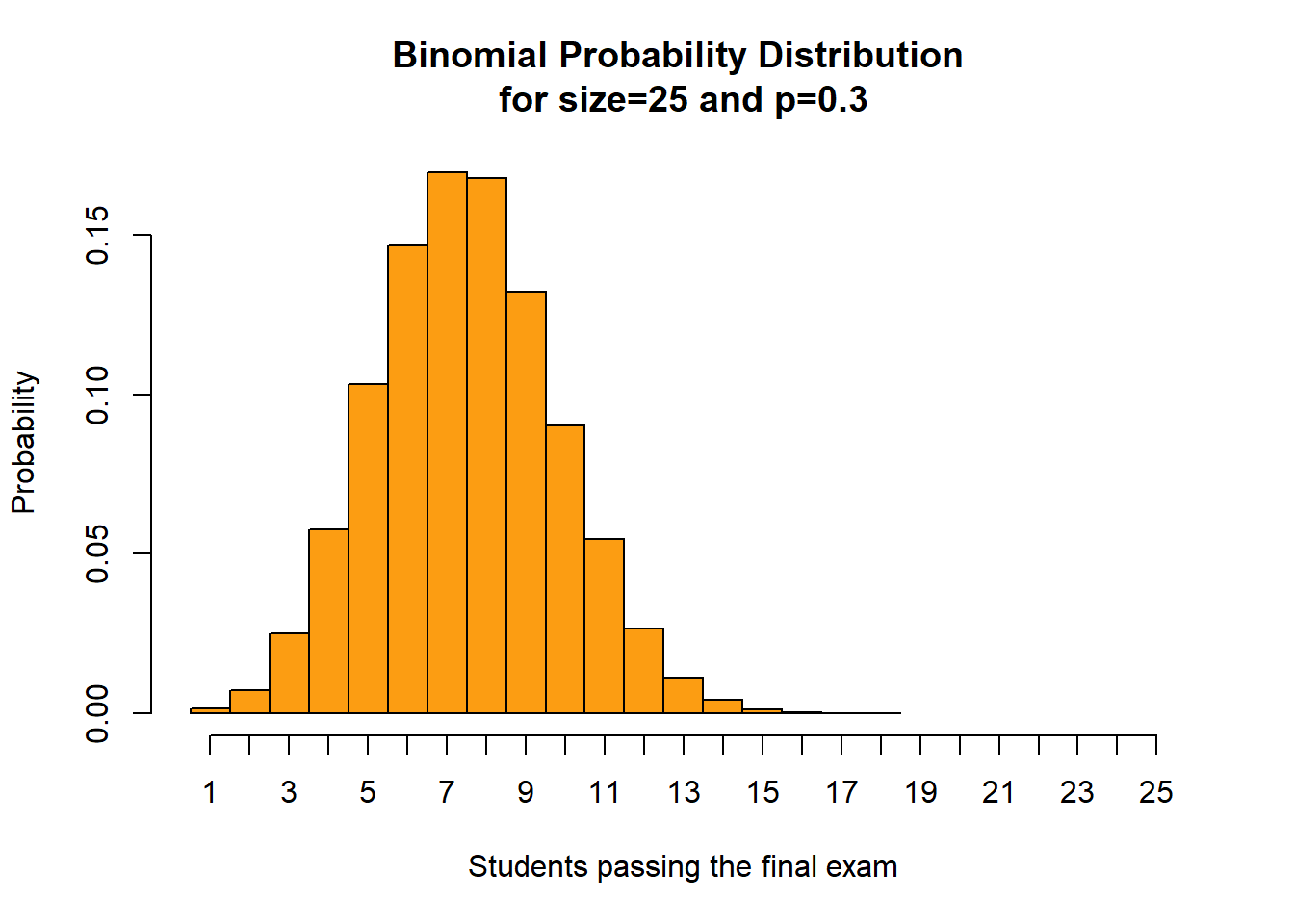In addition, we visualize the discussed probabilities from above: $$P(X = 3)$$, $$P(X = 15)$$, $$P(X \le 9)$$, $$P(X > 9)$$ (upper panel) and the corresponding cumulative binomial probability distribution function (lower panel).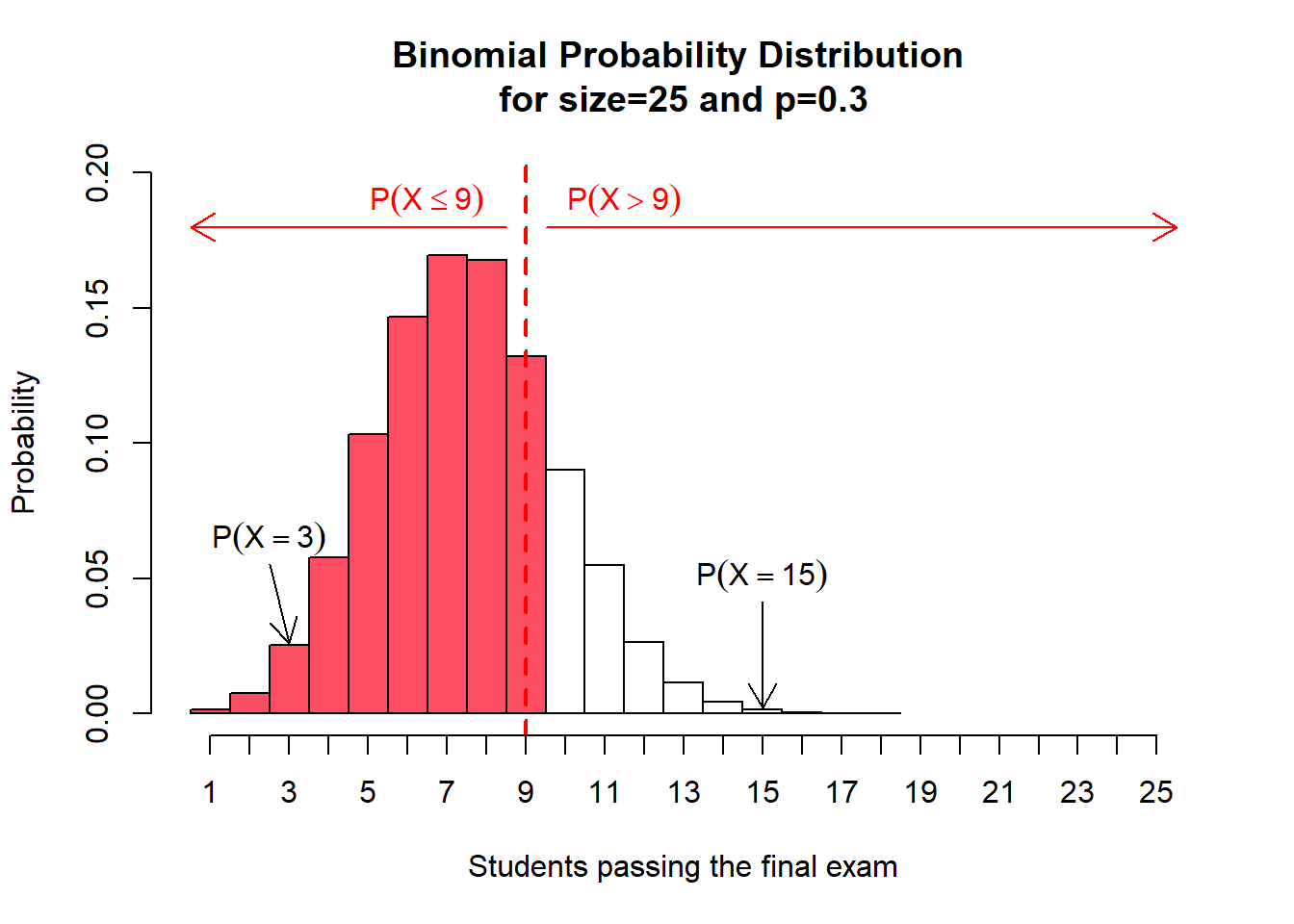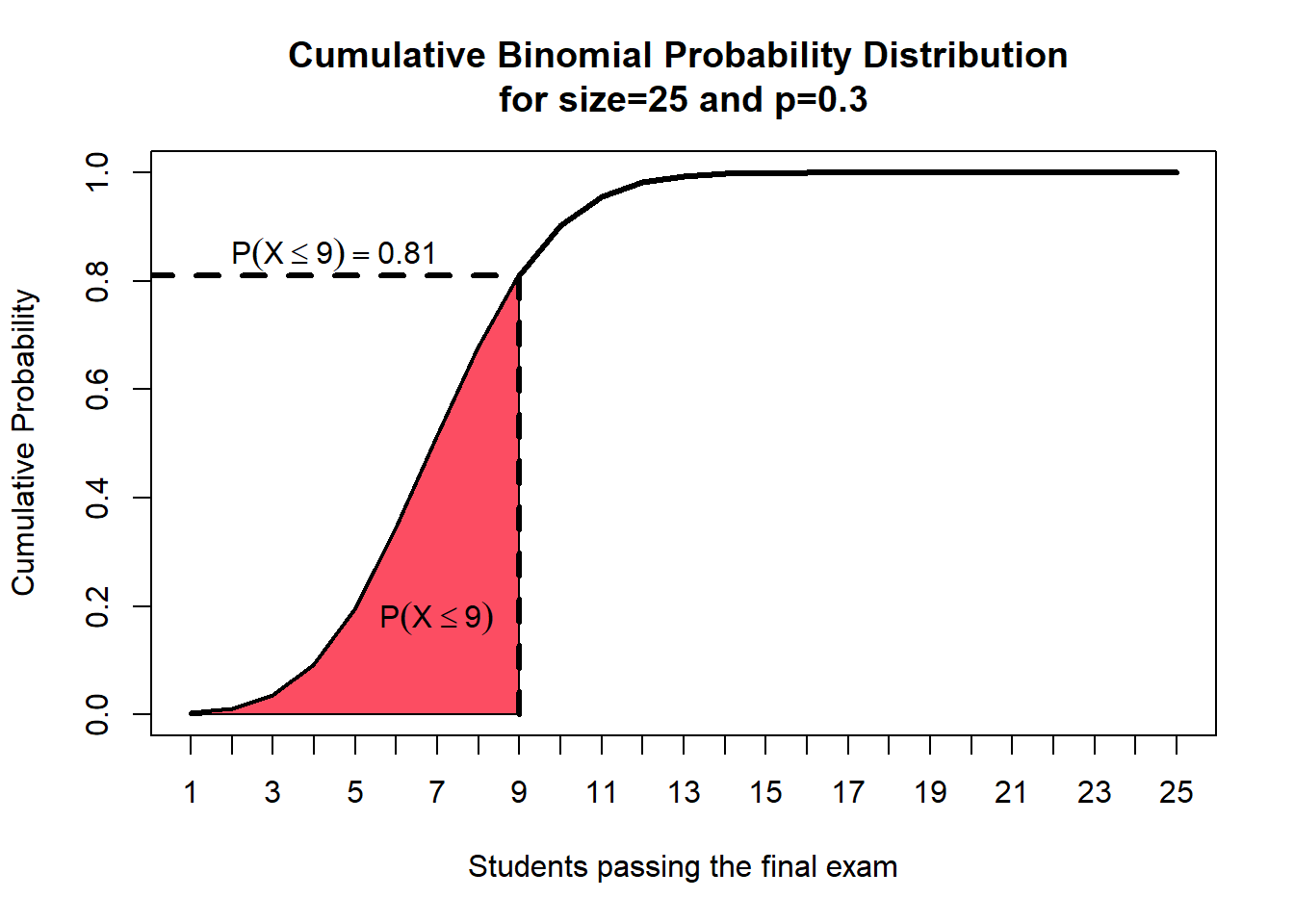In order to conclude this section and in order to give you some intuition of the shapes of different binomial probability distributions, three different binomial probability distributions and their corresponding cumulative binomial probability distributions for $$p=0.1$$, $$p=0.5$$, $$p=0.9$$ are given below.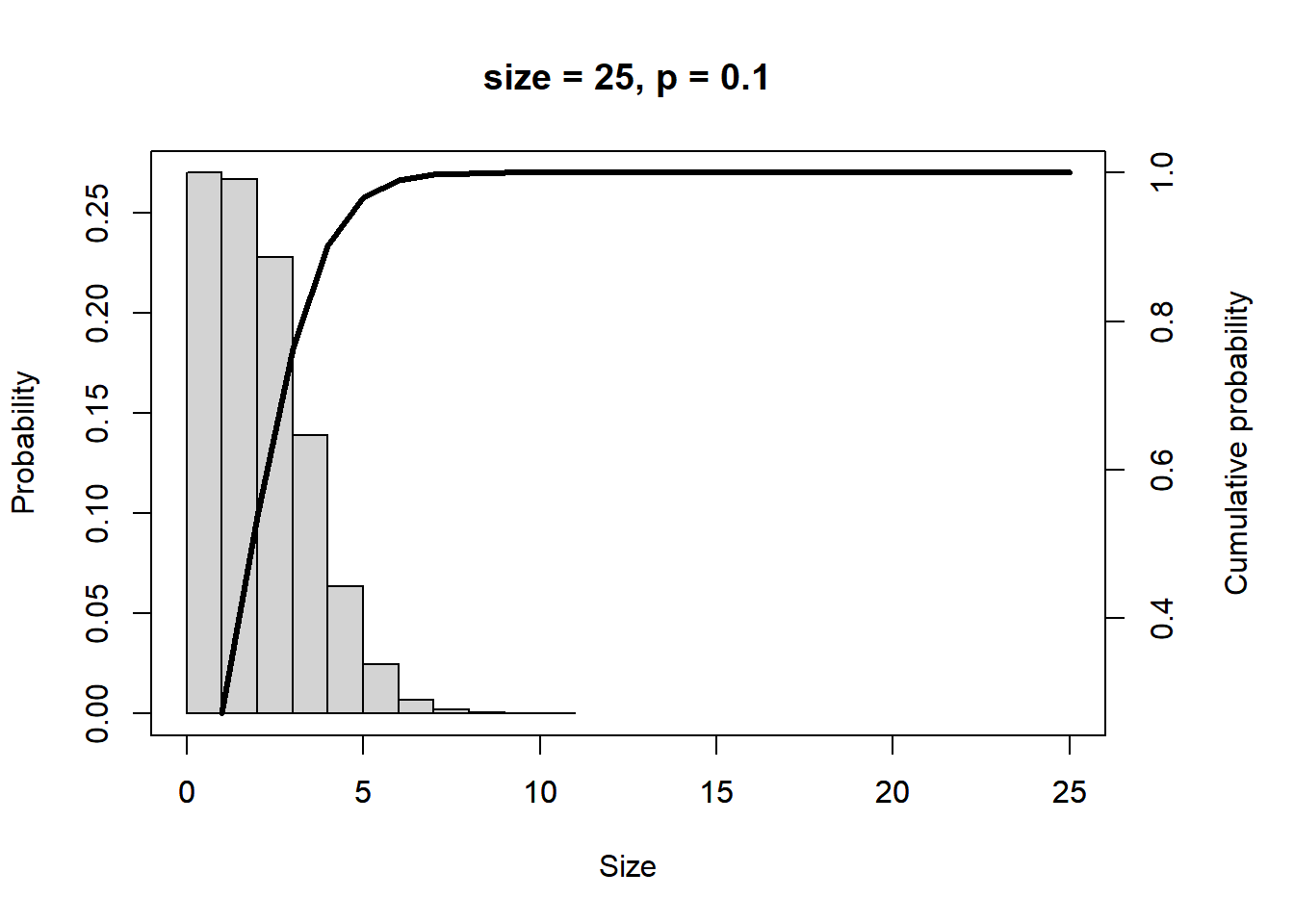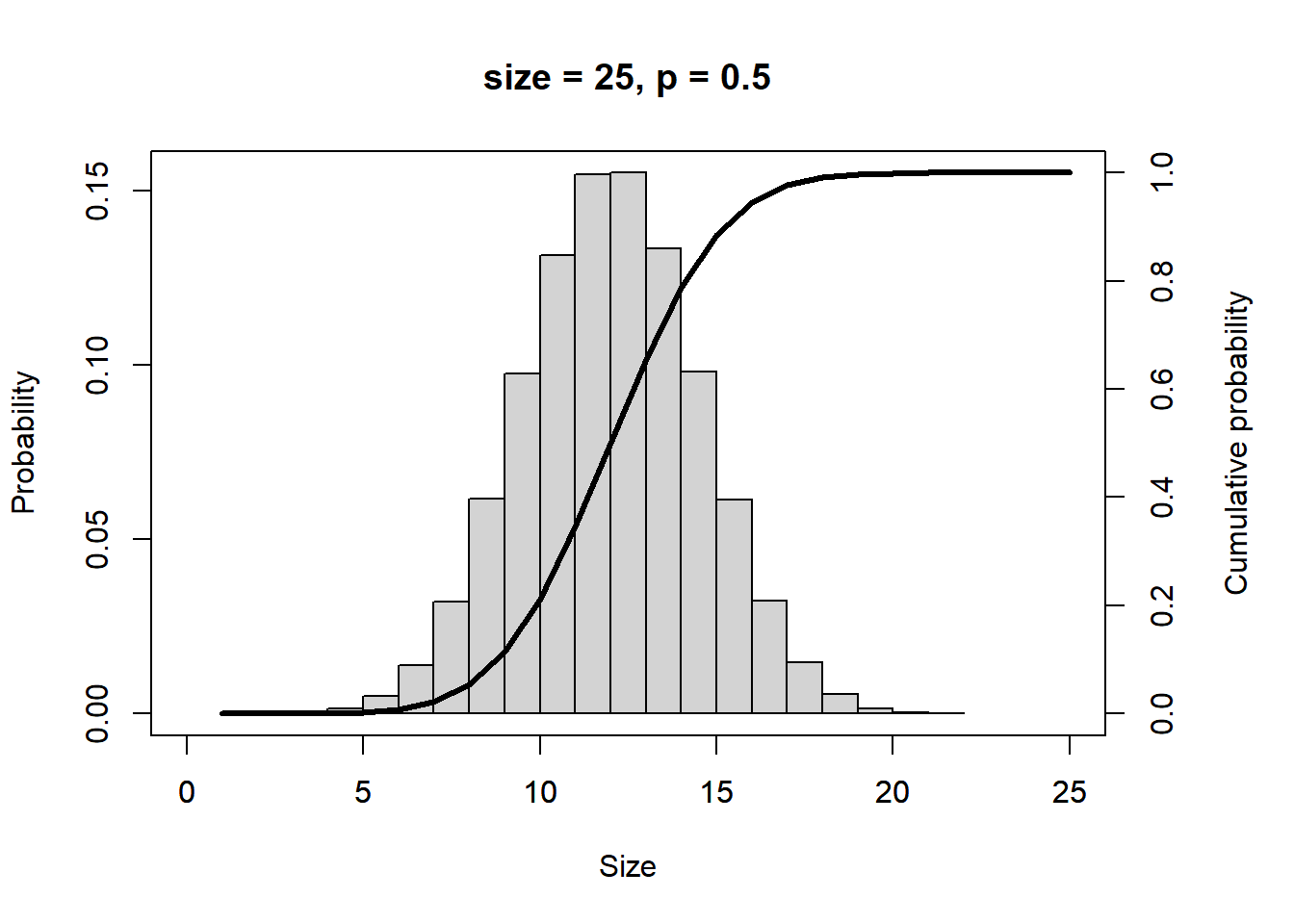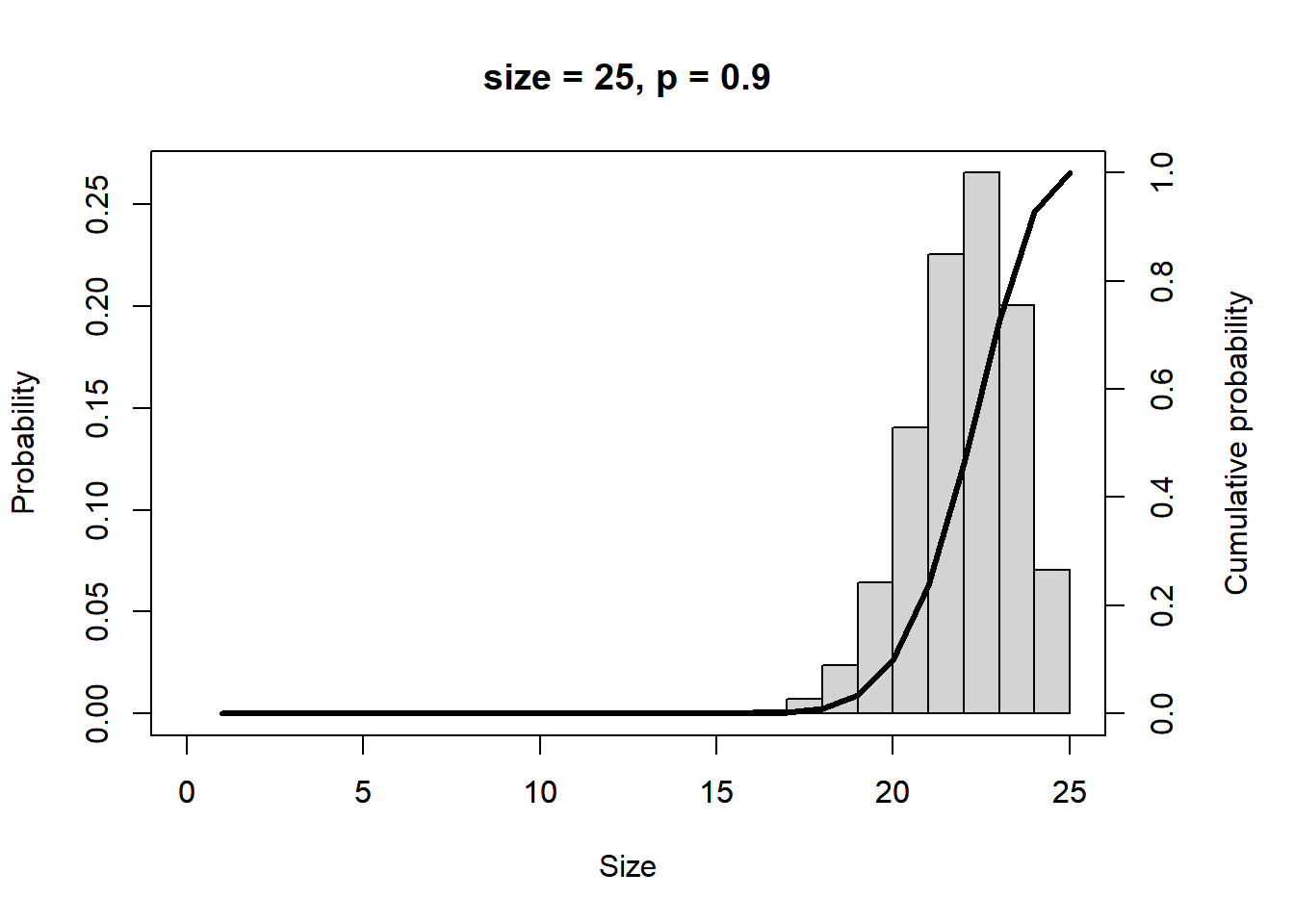Exercise: Imagine we found hints for 3 twenty-year-flood events (HQ20) within a 10 year time frame in a historical archive. Calculate the probability for this! Note: A HQ20 indicates a flood with a probability of p = 1/20 = 0.05 for a certain year.

### your code here
Show code
p <- 1 / 20
n <- 10

paste("The probability for three HQ20 in a decade is:", dbinom(x = 3, size = n, prob = p))
##  "The probability for three HQ20 in a decade is: 1.05 %"

Advanced Exercise: How many twenty-year-flood events may occur per decade with a probability of more than 98 %? Hint: Have a look at R’s qbinom() function!

### your code here
Show code
p <- 1 / 20
n <- 10

paste("With a probability of 98 %", qbinom(p = 0.95, size = n, prob = p), "or less twenty-year-flood events occur per decade.")
##  "With a probability of 98 % 2 or less twenty-year-flood events occur per decade."

Citation

The E-Learning project SOGA-R was developed at the Department of Earth Sciences by Kai Hartmann, Joachim Krois and Annette Rudolph. You can reach us via mail by soga[at]zedat.fu-berlin.de.You may use this project freely under the Creative Commons Attribution-ShareAlike 4.0 International License.

Please cite as follow: Hartmann, K., Krois, J., Rudolph, A. (2023): Statistics and Geodata Analysis using R (SOGA-R). Department of Earth Sciences, Freie Universitaet Berlin.